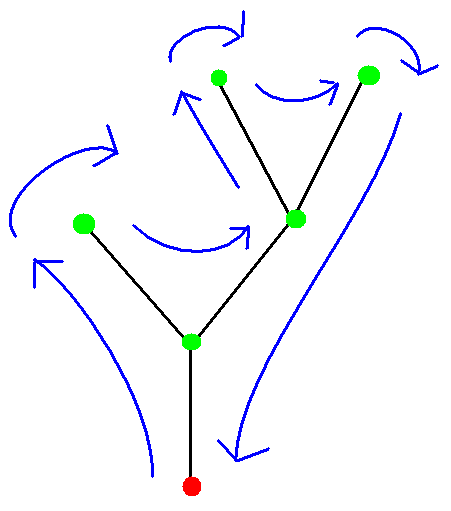## August 2012 riddle

This riddle is an invention of my own. I hope you like it.

Let T be a finite rooted tree. ("Finite" means that it has finitely many vertices, "rooted" means that one of the vertices has been designated the tree's root. In a rooted tree, there is a clear parent/offspring relation between vertices, where the "offspring" of a vertex are the vertices adjacent to it that are farther away from the root and its "parent" is the vertex adjacent to it that is closer to the root.)

The figure below shows an example of such a tree, in black. The vertices are in green, except for the root, which is in red. (Note: the tree in the example is a binary tree, but the question deals with general trees that are finite and rooted.)(a) (b)

To be exact, both drawings (a) and (b) are of the same tree. They are different embeddings of the tree in 2D space. The tree itself has no notion for the order of the vertices, so the short branch can be depicted as either to the left or to the right.

In blue, you see a path going around each of the embeddings. Such a path, keeping the tree to its right at all times, is known as an Eulerian tour of the tree. Let us define the Euler number of a tree embedding as follows.

Take the Eulerian tour. Each time the path goes to an offspring, write a "1". Every time it goes to a parent, write a "0". The Euler number of an embedding is the number that you get, when preceded by "0.", where the period is a decimal point, and the number is in binary. For the two examples shown here, we have e(a)=0.1101101000BIN and e(b)=0.1110100100BIN. Obviously, the trailing zeroes do not change the value of the number.

So far, these are only Euler numbers for embeddings. The Euler number of a tree is the maximum among all Euler numbers for all its embeddings. In the example: E(T)=0.1110100100BIN.

This month the riddle has two parts. Answer both for credit.

#### Part 1:

The set of all Euler numbers for all tree embeddings is a subset of the numbers between 0 and 1. The question is: is this set well ordered? Prove your answer.

To clarify: a "well ordered" set is a set for which every subset, even a subset of infinite size, necessarily has a minimum (and not only an infimum). For example, the set of all positive integers is well ordered, but the set of all reals between 0 and 1 is not. If we take (as an example) a subset of the reals between 0 and 1 that includes all reals greater than 0.5, this subset has 0.5 as its infimum, but it has no minimum, because 0.5 is not part of the set.

#### Part 2:

Is the set of all numbers that are Euler numbers for some tree a well ordered set? Again, prove your answer.

### List of solvers:

Lu Wang & Jiongxin Jin (3 August 19:58)
Oded Margalit (5 August 21:14)
Jim Boyce (7 August 10:10)
Elegant and original solutions can be submitted to the puzzlemaster at riddlesbrand.scso.com. Names of solvers will be posted on this page. Notify if you don't want your name to be mentioned.